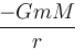Courses

# Quantum Mechanics In Three Dimensions MCQ

## 10 Questions MCQ Test Topic wise Tests for IIT JAM Physics | Quantum Mechanics In Three Dimensions MCQ

Description
This mock test of Quantum Mechanics In Three Dimensions MCQ for Physics helps you for every Physics entrance exam. This contains 10 Multiple Choice Questions for Physics Quantum Mechanics In Three Dimensions MCQ (mcq) to study with solutions a complete question bank. The solved questions answers in this Quantum Mechanics In Three Dimensions MCQ quiz give you a good mix of easy questions and tough questions. Physics students definitely take this Quantum Mechanics In Three Dimensions MCQ exercise for a better result in the exam. You can find other Quantum Mechanics In Three Dimensions MCQ extra questions, long questions & short questions for Physics on EduRev as well by searching above.
QUESTION: 1

### A three dimensional harmonic oscillator is in thermal equilibrium with a temperature reservoir at temperature T. The average total energy of the oscillator is :

Solution:

Average K.E. in one dimension is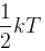Average K.E. in 3 dimension is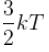The correct answer is: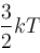QUESTION: 2

### Consider the particle in a three dimensional box problem for 2 boxes. One having equal edges of length a  and the other of edges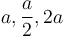along  x, y and z  coordinates respectively.

Solution: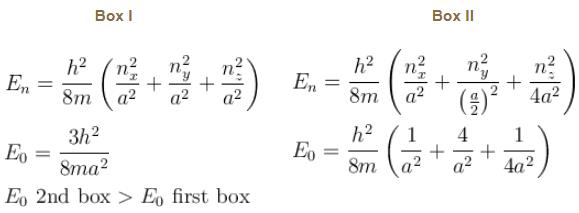The correct answer is: Ground state energy of the second box is greater

QUESTION: 3

### For the 3-dimensional second box:

Solution:

First excited state, (nxnynz) can be (1, 1, 2) or (1, 2, 1) or (2, 1, 1)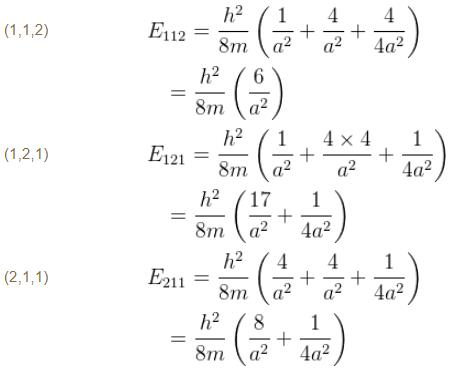Now two energy levels have the same energy. Hence non degenerate
The correct answer is: The first excited state is non degenerate

QUESTION: 4

What will be quantum number ‘n’ for earth? Take the earth sun distance to be 1.496 × 1011m

Solution:

Energy levels for hydrogen atom are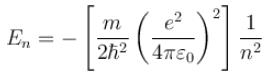Again substituting for the gravitational analog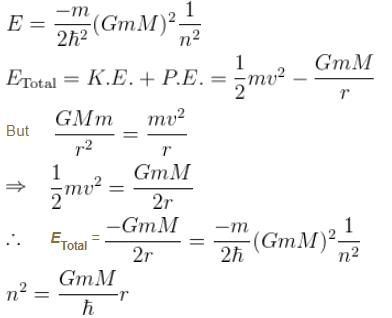here r is the earth sun distance
r = 1.496 × 1011m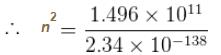n = 2.53 × 1074
The correct answer is: n ~ 1074

QUESTION: 5

Which of the following is true about a quantum harmonic oscillator?
(1) A spectrum of evenly spaced energy states
(2) A potential energy function that is linear in the position coordinate
(3) A ground state characterized by zero kinetic energy.
(4) A non zero probability of finding the oscillator outside the classical turning points.

Solution:

A quantum harmonic oscillator: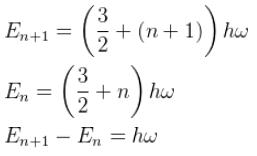∴  Equally spaced

Potential Energy function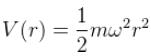For ground state,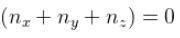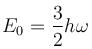A quantum Harmonic oscillator has non zero probability of being found beyond the classical turning points! This is one of the basic difference between a classical & quantum Harmonic oscillator

The correct answer is: 1 and 4 only

QUESTION: 6

Which of the following functions could represent the radial wave function for an electron in an atom? (r is the distance of the electron from the nucleus; Ab are constants)

I. Aebr
II. A sinbr
III. A/r

Solution:

The wave function of an electron should satisfy the following properties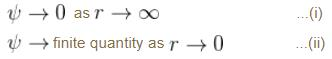The only function (from the ones given) that satisfies these conditions is Ae–br

The correct answer is: I only

QUESTION: 7

Suppose the earth made a transition to the next lower level (n – 1). How much energy (in Joules) would be released? What would be the wavelength of the emitted photon be?

Solution: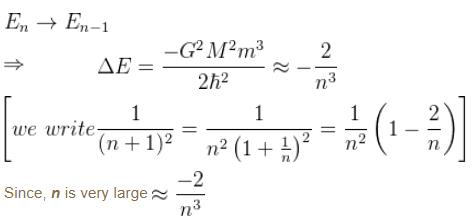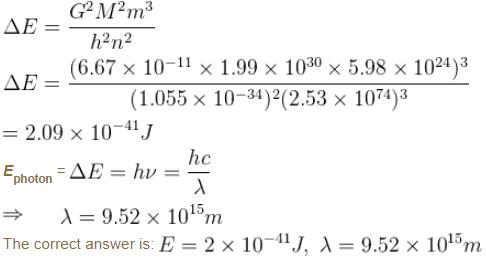QUESTION: 8

Box I has each edge of length ‘a’ units. Box II has edges as a, 2a, a/2 along the xy and z axis respectively. For what values of nxnynz will the first excited state energy of box II be same as the first excited state energy of Box I

Solution: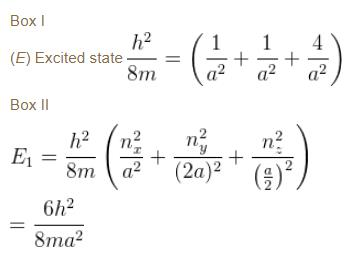For nx - 1, ny = 2, nz = 1
(E1) box II  = (E1) box I
The correct answer is: (nxnynz) is (1, 2, 1)

QUESTION: 9

What will be the Bohr radius of this system (work out the actual numerical value)

Solution: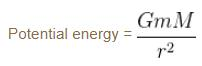In the case of hydrogen atom,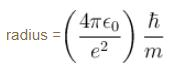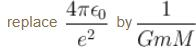for the gravitational analog.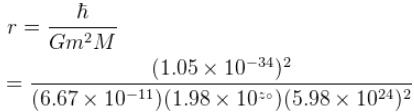= 2.34 × 10–138m
The correct answer is: r ~ 2 × 10–138m

QUESTION: 10

Consider the Earth-Sun system as a gravitational analog to the hydrogen atom.

What is the potential energy function. (Let m be the mass of earth and M be the mass of the sun)

Solution: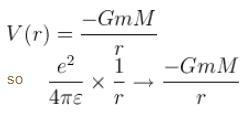transforms hydrogen results to the gravitational analogs
(–)ve  sign for attractive force.

The correct answer is: